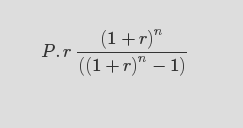Diesel vs Petrol CAR
Which Car to buy? Confused? This simple tool might help! Change values to your requirements and decide.

### DIESEL CAR

YearTotal expenses(Petrol)Total expenses(Diesel)

Note: This comparison doesn't include the variation in fuel prices. these values are provided for indicative purpose only.

## How it works?

Car price = Cash in hand + Loan amount

Less loan amount and less EMI if Petrol Car,
Petrol loan amount = (Loan amount - (diesel car price-petrol car price))

Loan interest paid = (EMI * n) - Loan amount
where n is loan term in months

Total Car price = Onroad price + Loan interest paid

Fuel price per year = (monthly average * 12 * price per ltr) / mileage

Total expenses till yth year = Total Car price + Fuel price per year * y

## How to calculate EMIWhere:
P = Principal amount(Loan amount),
r = Rate of interest(if interest is 12%, then rate of interest is 12/12/100 = 0.01)
n = Term in months